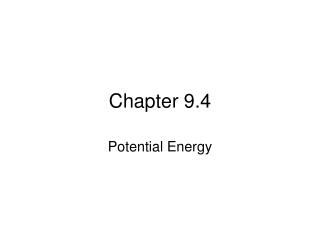Download PresentationChapter 9.4

# Chapter 9.4

Download Presentation## Chapter 9.4

- - - - - - - - - - - - - - - - - - - - - - - - - - - E N D - - - - - - - - - - - - - - - - - - - - - - - - - - -
##### Presentation Transcript

1. Chapter 9.4 Potential Energy

2. 9.4Potential Energy Energy that is stored and held in readiness is called potential energy (PE) because in the stored state it has the potential for doing work.

3. 9.4Potential Energy Three examples of potential energy • gravitational potential energy. • elastic potential energy • chemical (potential) energy

4. 9.4Potential Energy Gravitational Potential Energy • Energy is stored in an object as the result of increasing its height. • Work is required to elevate objects against Earth’s gravity. • Example: Water in an elevated reservoir and the raised ram of a pile driver have gravitational potential energy.

5. 9.4Potential Energy Elastic Potential Energy—potential to do work • Energy stored in a stretched or compressed spring or material. • When a bow is drawn back, energy is stored and the bow can do work on the arrow. • These types of potential energy are elastic potential energy.

6. CHEMICAL POTENTIAL ENERGY • Energy due to the bond position between molecules (stored during bonding). • Potential chemical energy is released from chemical reactions (burning, for example). • Fuels, Food, Batteries, for example.

7. 9.4Potential Energy Chemical Energy The chemical energy in fuels is also potential energy. It is energy of position at the submicroscopic level. This energy is available when the positions of electric charges within and between molecules are altered and a chemical change takes place.

8. 9.4Potential Energy The amount of gravitational potential energy possessed by an elevated object is equal to the work done against gravity to lift it. PE = mgh What is the gravitational PE of a 10.0 kg object at 4.00 m above the ground? mg is weight (in newtons) [mass (kg) x gravity (m/s2)] 10 kg x 9.8 m/s2 x 4 m = 392 J

9. 9.4Potential Energy • The potential energy of the 100-N boulder with respect to the ground below is 200 J in each case. • The boulder islifted with 100 N of force.

10. 9.4Potential Energy • The potential energy of the 100-N boulder with respect to the ground below is 200 J in each case. • The boulder islifted with 100 N of force. • The boulder ispushed up the 4-m incline with 50 N of force.

11. 9.4Potential Energy • The potential energy of the 100-N boulder with respect to the ground below is 200 J in each case. • The boulder islifted with 100 N of force. • The boulder ispushed up the 4-m incline with 50 N of force. • The boulder islifted with 100 N of force up each 0.5-m stair.

12. 9.4Potential Energy think! You lift a 100-N boulder 1 m. a. How much work is done on the boulder? b. What power is expended if you lift the boulder in a time of 2 s? c. What is the gravitational potential energy of the boulder in the lifted position?

13. 9.4Potential Energy think! You lift a 100-N boulder 1 m. a. How much work is done on the boulder? b. What power is expended if you lift the boulder in a time of 2 s? c. What is the gravitational potential energy of the boulder in the lifted position? Answer: a. W = Fd = 100 N·m = 100 J b. Power = 100 J / 2 s = 50 W c. Relative to its starting position, the boulder’s PE is 100 J. Relative to some other reference level, its PE would be some other value.

14. 9.4Potential Energy Name three examples of potential energy.